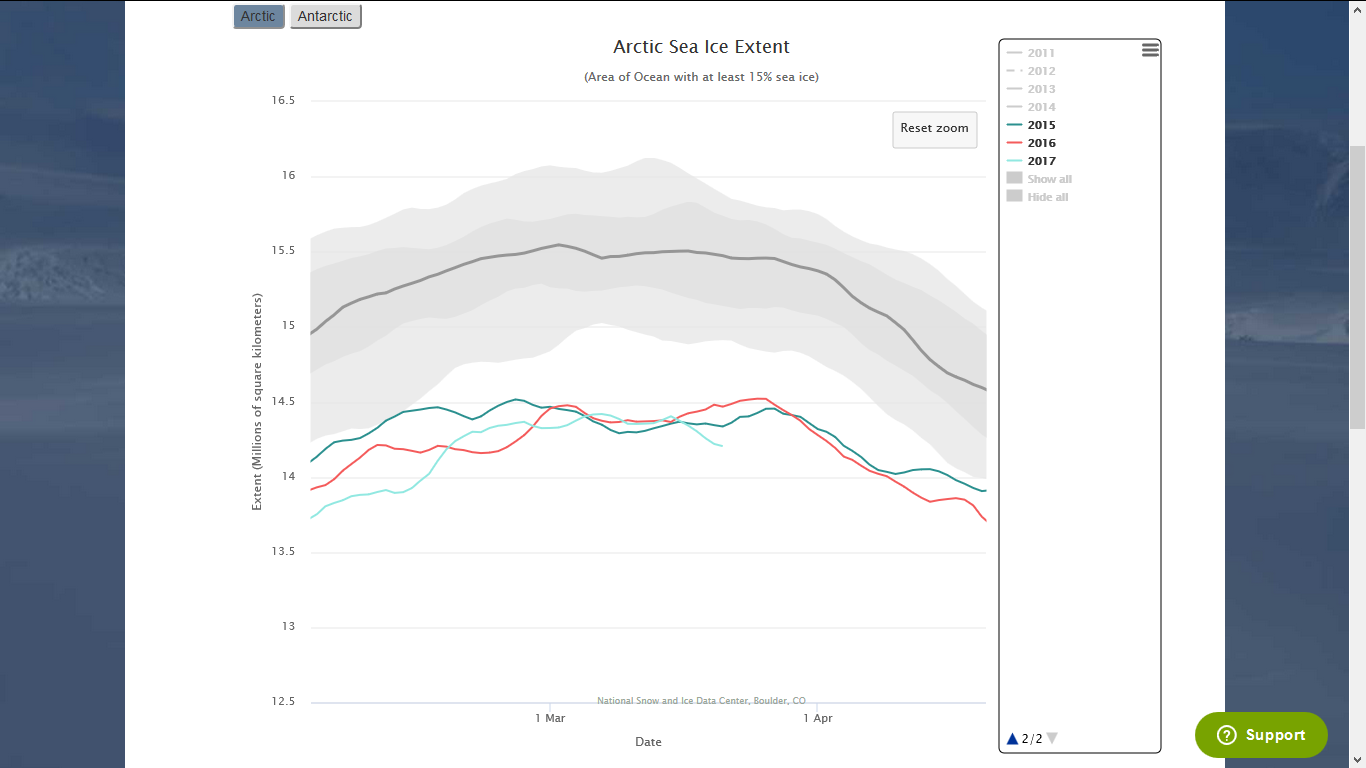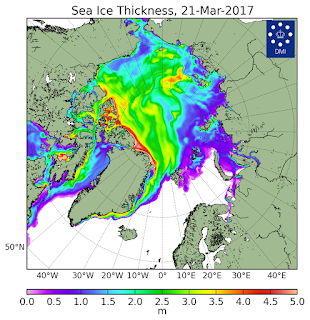## Wednesday, March 22, 2017

### 2017 Arctic Sea Ice Melt Season Has Started

The National Snow and Ice Data Center (NSIDC) has not yet announced the Arctic sea ice maximum extent for this year, but I expect them to do so momentarily. (UPDATE: NSIDC made the announcement mere hours after I wrote this.) And, I expect them to announce the maximum extent for 2017 was 14.420 million square kilometers and occurred on March 7. When this is confirmed, it will be the lowest maximum extent ever recorded and 2017 will be the third year in a row to experience the record low maximum. (UPDATE: You can read a nice article about the changes in the sea ice here.) The graphic below shows the sea ice extent for 2017 (light blue), 2016 (red), 2015 (dark blue) and the 1981-2010 median extent (dark grey). The shaded areas are one and two standard deviations from the median.Source: NSIDC
This means the 2017 Arctic sea ice melt season is now underway and, considering the terrible shape of the maximum extent, we already have to be concerned about the minimum extent coming in September. Given this situation, I decided to make an attempt at a very early prediction on the minimum. I predicted the maximum would be 14.2 million square kilometers and would occur in the first week of March, so I was pretty close.

One of the dire situations that doesn't show up in the above graph is the ice thickness, which is in even worse shape than the extent. This is a plot of the ice thickness from the Polar Portal.Source: Polar Portal
I expect all ice 2 meters thick and thinner to completely melt by September. That is the ice colored light blue to purple and violet. Likewise, I expect all of the ice 3.5 meters thick and thicker to survive, albeit in a much thinner condition. That would be the yellow, red and white colored areas. The green areas are a toss-up. A great deal of the melting depends on the weather in the Arctic region during the summer. If the summer is cloudy and cold, more of the green area will survive. If the summer is clear and sunny, more will melt. I used a figure of 50% melt for the green areas in my estimate. That only leaves adding up the areas, which really wasn't all the difficult. The Polar Portal conveniently included some marked blocks. I merely had to find the area of the marked areas.

The surface area of a part of a globe is called a spherical cap and the math is straight forward. I have included a description of the math below. These calculations gave me 3.9 million square kilometers for the area inside the most poleward dotted circle. I used an even four million for my estimate. The circle indicates 80 degrees north. The next larger dotted circle indicates 70 degrees north. This area is also divided up into 10 degree-wide blocks. I calculated the area of each block to be 323,000 square kilometers and I rounded up to 325,000.

I estimate one half of the area of the 80 circle to still have ice in September (even including the land area as 'ice free'). This is 2 million square kilometers. Of the 10-degree squares, I expect there will be only three blocks worth of ice remaining at the minimum. That is another 975,000 square kilometers. Let's call it an even one million.

So, my very early forecast is for the 2017 minimum extent to be about 3 million square kilometers. This would be the lowest minimum extent ever recorded and would easily shatter the previous record low of 3.387 million square kilometers set in 2012.

Of course, we'll have to wait until September to see how well I do with this forecast. Unfortunately, I feel that my estimate is conservative and fear the actual extent will be even lower. Let's hope I'm wrong.

MATH USED

A spherical cap is the surface area of a sphere cut off by a plane. If the plane cuts the sphere in half, the spherical cap is called a hemisphere.

The area of a cap is found by the equations

A = 2πrh  = π(r2 + a2)

A = the area of the cap
r = the radius of the sphere
h = the height of the cap
a = the radius of the cap

r = 6400 kilometers (one Earth radii)

θ = 10o (90o - 80o) for the 80o circle and 20o (90o - 70o) for the 70o circle
a = r sinθ = (6400 km)sin10o = 1111 km (80o circle)
and
a = r sinθ = (6400 km)sin20o = 2189 km (70o circle)
h = r - rcosθ = 6400 km - (6400 km)cos10o = 97 km (80o circle)

and
h = r - rcosθ = 6400 km - (6400 km)cos20o = 386 km (70o circle)

The area of the 80o circle is simple and is merely 2π(6400 km)(97 km) = 3.9 million square km
The area of the 70o circle is 2π(6400 km)(386 km) = 15.5 million square km

If you use the alternative equation as a check, you get the same results.

However, we don't want the surface area of the 70o circle, we want the area outside of the 80o circle, so we need to subtract the area of the smaller circle from the larger one and this is equal to 11.6 million square km. This is the area of the band between the 70o circle and the 80o circle. This area is divided up into 36 10-degree-wide blocks, giving us 323,000 square kilometers per 10o x 10o block on the Polar Portal graphic.